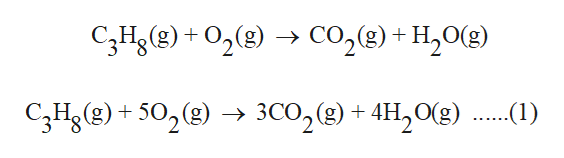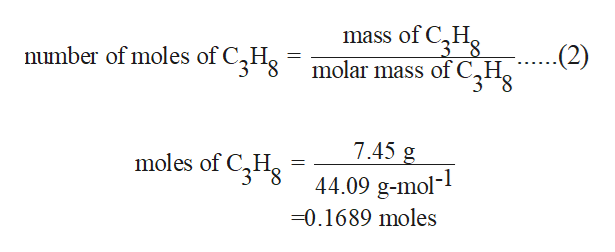11. Propane (C3H8) burns in oxygen to produce carbon dioxide gas and water vapor:(a) Write a balanced equation for this reaction. (b) Calculate the number of liters of carbon dioxide measured at STP that could be produced from 7.45 g of propane.

Question

11. Propane (C3H8) burns in oxygen to produce carbon dioxide gas and water vapor:
(a) Write a balanced equation for this reaction.
(b) Calculate the number of liters of carbon dioxide measured at STP that could be produced from 7.45 g of propane.

Step 1

The unbalanced chemical reaction is as follows:

The above reaction can be balanced by balancing the number of atoms of each element on both sides of the given reaction. The balanced chemical reaction should follow the law of conservation of mass.

The balanced chemical reaction is as follows:

Equation (1) represents the required balanced equation.help_outlineImage TranscriptioncloseC3H)02()Co2(8) + H2O{g) 3CO2 (g)+4H20g) C3Hg(g)502(g) -> (1) fullscreen
Step 2

From the balanced equation (1), it is evident that 1 mole of C3H8 produces 3 moles of CO2.

Number of moles of C3H8 can be calculated by the use of formula given below as,

Mass of C3H8 is 7.45 g.

Molar mass of C3H8 is 44.09...help_outlineImage Transcriptionclosemass of CH 3**8 mumber of moles of C3Hg molar mass of C,Hg 7.45 g moles of CHg 44.09 g-mol- 0.1689 moles fullscreen

Want to see the full answer?

See Solution

Want to see this answer and more?

Our solutions are written by experts, many with advanced degrees, and available 24/7

See Solution
Tagged in

Chemistry